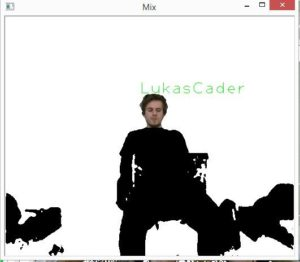Posted on

# Face recognition using depth data from Kinect sensor

We will segment face from color camera with use of depth data and run recognition on it using OpenCV functions: EigenFaces, FisherFaces and LBPH.

Complete process is as follows:

1. First we need to obtain RGB and depth stream from Kinect sensor and copy it to byte array in order to be usable for OpenCV
```IColorFrame* colorFrame = nullptr;
IDepthFrame* depthFrame = nullptr;
ushort* _depthData = new ushort[depthWidth * depthHeight];
byte* _colorData = new byte[colorWidth * colorHeight * BYTES_PER_PIXEL];

colorFrame->CopyConvertedFrameDataToArray(colorWidth * colorHeight * BYTES_PER_PIXEL, _colorData, ColorImageFormat_Bgra);
depthFrame->CopyFrameDataToArray(depthWidth * depthHeight, _depthData);

```
2. Because color and depth camera have different resolutions we need to map coordinates from color image to depth image. (We will use Kinectâ€™s Coordinate Mapper)
```m_pCoordinateMapper->MapDepthFrameToColorSpace(depthWidth * depthHeight,(UINT16*) _depthData, depthWidth * depthHeight, _colorPoints);
```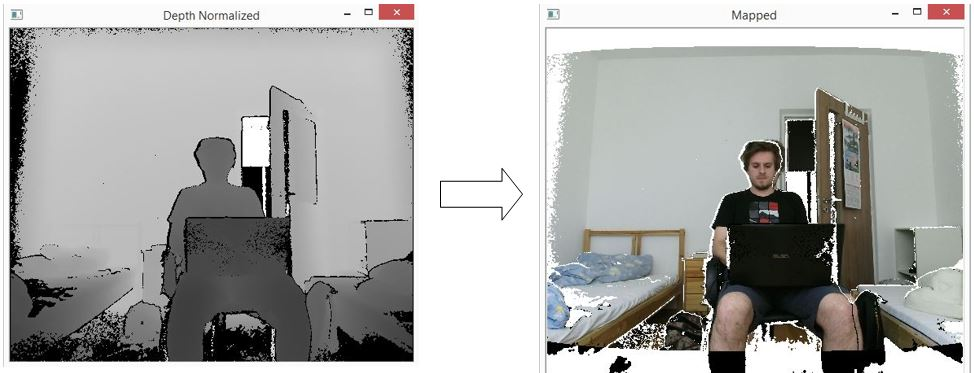3. Because we are going to segment face from depth data we need to process them as is shown in the next steps:
1. Unmodified depth data shown in 2D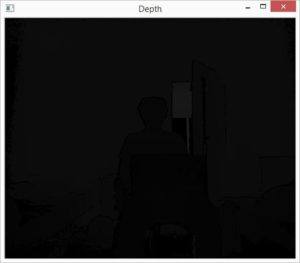2. Normalization of values to 0-255 range
â€“Â Better representation

```cv::Mat img0 = cv::Mat::zeros(depthHeight, depthWidth, CV_8UC1);
double scale = 255.0 / (maxDist - minDist);
depthMap.convertTo(img0, CV_8UC1, scale);
```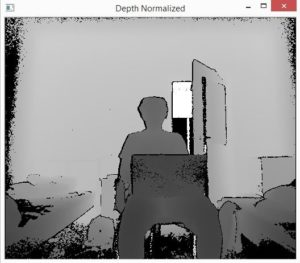3. Removal of the nearest points and bad artifacts
â€“Â the points for which Kinect canâ€™t determine depth value are by default set to 0 â€“ we will set them to 255

```if (val < MinDepth)
{
image.data[image.step * i + image.step * j + 0] = 255;
}

```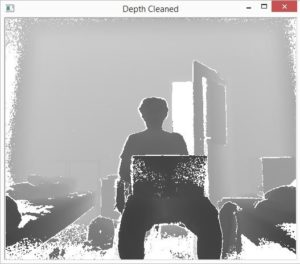4. Next we want to segment person, we apply depth threshold to filter only nearest points and the ones within certain distance from them and apply median blur to image to remove unwanted artifacts such as isolated points and make edges of segmented person less sharp.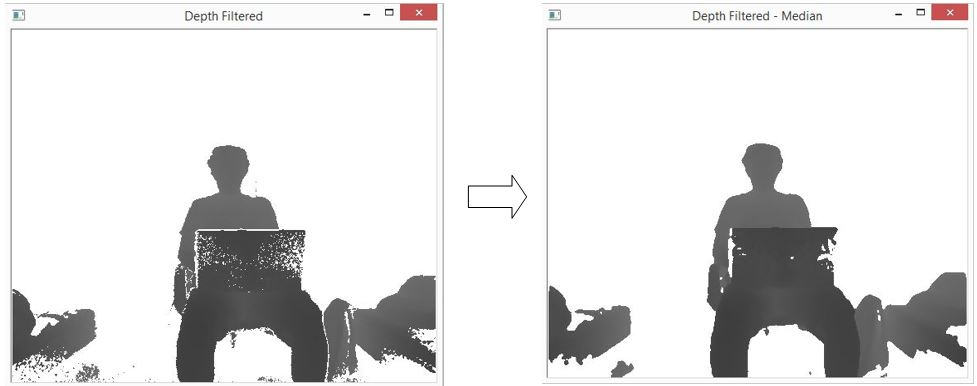```if (val > (__dpMax+DepthThreshold))
{
image.data[image.step * i + image.step * j + 0] = 255;
}
```
4. Now when we have processed depth data we need to segment face. We find the highest non-white point in depth map and mark it as the top of head. Next we make square segmentation upon depth mask with dynamic size (distance from user to sensor is taken into account) from top of the head and in this segmented part we find the leftmost and rightmost point and made second segmentation. The 2 new points and point representing top of the head will now be the border points of the new segmented region. (Sometimes because of dynamic size of square we have also parts of shoulders in our first segmentation, in order to mitigate this negative effect we are looking for leftmost and rightmost point only in the upper half of the image)
```if (val == 255 || i > (highPointX + headLength) || (j < (highPointY - headLength / 2) && setFlag) || (j > (highPointY + headLength / 2) && setFlag))
{
//We get here if point is not in face segmentation region
...
}
else if (!setFlag)
{
//We get here if we find the first non-white (highest) point in image and set segmentation region
highPointX = i;
highPointY = j;
headLength = 185 - 1.2*(val); //size of segmentation region
setFlag = true;
...
}
else
{
//We get here if point is in face segmentation region and we want to find the leftmost and the rightmost point

if (j < __leftMost && i < (__faceX + headLength/2)) __leftMost = j;
if (j > __rightMost && i < (__faceX + headLength/2)) __rightMost = j;
}

```
5. When face is segmented we can use one of OpenCV functions for face recognition and show result to the user.